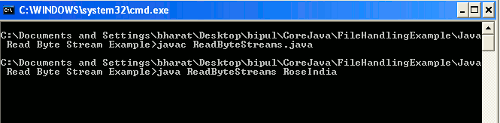# Java Byte Streams Example

In this section we will discuss how to read one byte at a time from the input stream.## Java Byte Streams Example

In this section we will discuss how to read one byte at a time from the input stream.

To read the input stream as byte streams Java provided an abstract class named InputStream through the java.io package. All the classes that represents the input stream of bytes are the subclasses of InputStream. In this example I have used the ByteArrayInputStream class which has the internal buffer which keeps the bytes which may be read from the stream. The close() method of this class has no effect i.e. after closing of ByteArrayInputStream methods in this class can be called without throwing an IOException.

There are two constructors of this Class :

 Constructor Name Description ByteArrayInputStream(byte[] buf) Using this constructor a ByteArrayInputStream is created which takes an array as argument for its buffer array. ByteArrayInputStream(byte[] buf, int offset, int length) Using this constructor a ByteArrayInputStream is created which takes an array as argument for its buffer array with the specified offset and length.

Methods of ByteArrayInputStream

Example :

Here an example is being given which will demonstrate how to read one byte from the input stream at a time. In this example I have created a class named ReadByteStreams with the public static void main(String args[]) method. Using this class I have tried to take the String input through command line and then converted this String to a byte array. Then created an instance of InputStream and used the constructor of ByteArrayInputStream(byte[] b) which takes the argument of byte for its buffer array. Then used the read() method of ByteArrayInputStream to read the input stream as byte. Here I am giving the code of this example below :

```/* Java Byte Streams Example
This example demonstrates how to
read one byte from the input stream at a time. */

import java.io.InputStream;
import java.io.ByteArrayInputStream;
import java.io.IOException;

{
public static void main(String args[])throws IOException
{
String str = args;
byte b[] = str.getBytes();
InputStream bais = null;
bais = new ByteArrayInputStream(b);
int r;
while ((r = bais.read()) != -1)
{
System.out.print((char) r + " ");
}
bais.close();
}
}```

When you will execute the above code you will have to give an input as follows :When you will press the Enter after providing the value then the output will be as follows :